### Home > MC2 > Chapter 11 > Lesson 11.2.3 > Problem11-51

11-51.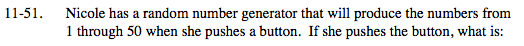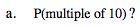Multiples of 10 are: 10, 20, 30, 40, and 50.

Since there are 50 possible outcomes and only 5 of those
outcomes are multiples of ten, what is the probability of
getting a multiple of 10?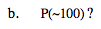The random number generator only produces numbers from
1 through 50. So what is the probability of not getting 100?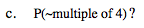Some multiples of 4 are: 4, 8, 12, 16, 20. Find the rest of
the multiples of 4 between 1 and 50.

There are 12 multiples of 4 between 1 and 50. So what is the
probability of NOT getting any of these 12 numbers?

$\frac{38}{50}$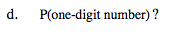How many one-digit numbers are between 1 and 50?

The only one-digit numbers are 1 through 9. So what is the
probability of getting a one-digit number?